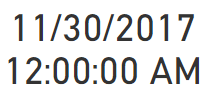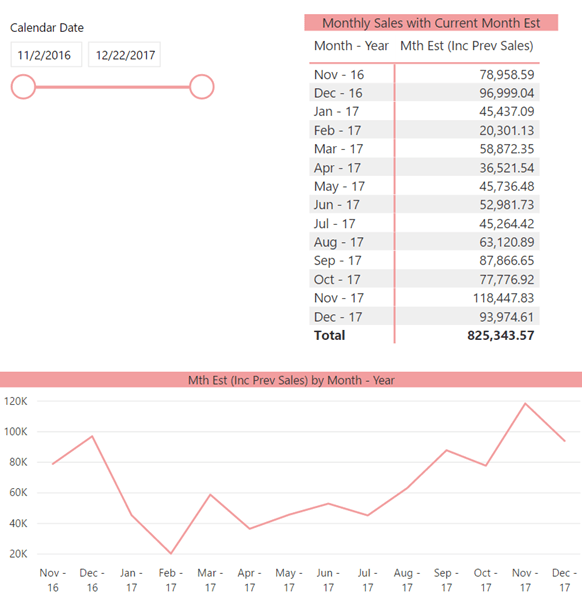Where I am consulting there was a requirement where for the current month they wanted to display the Current Month Estimate for the current month they were in, and to show the historical sales for all the previous months.

This makes some sound business sense, since currently month would typically start off with a lower sales amount, which would increase as each day goes by. Whilst the calculation for the Month Estimate is not very complex it does show if the sales are inline of where they should be.

In my example below, I am going to demonstrate how I did this, so that to the end user it appears to be one measure, even though I am using a few techniques for it to be displayed correctly.

## Working Example

In this example I have got some sales data, and I am going to demonstrate how to create the Month Estimate.

I will then create the [Sales Amount] Measure, and the [Current Month Estimate] Measure.

The [Current Month Estimate] is made up by this simple calculation:

• Sales for the current month I am in.
• Get the Days Gone in the current month I am in.
• Get the Total Days for the current month that I am in.
• For example, if my current date is 09 December 2017, then I would have the following details below.
• Sales for Current Month = \$36,819.60
• Days Gone in Current Month = 9
• Total Days in Current Month = 31

And then finally, I will show how create the magic measure, which will show the Sales up until the previous month (Nov 2017), and then for the current month (Dec 2017), it will display the measure [Sales Inc Est] which will be renamed to Sales Amount.

## Creating the standard measures

Below I created the following measures:

`Sales Amount = SUM('Orders'[Sales])`
```Days Gone in Curr Mth =
MAX ( 'Orders'[Order Date] )
RETURN
DAY (
)
```
```Days in Current Month =
MAX ( Orders[Order Date] )
VAR EndOfCurrMonth =
RETURN
DAY ( EndOfCurrMonth )
```
```Current Month Estimate =
DIVIDE ( [Sales Amount], [Days Gone in Curr Mth] ) * [Days in Current Month]
```
```Mth Est (Inc Prev Sales) =
MAX ( 'Orders'[Order Date] )
VAR Start12MonthsAgo =
DATE ( YEAR ( LastDataDate ) - 1, MONTH ( LastDataDate ) - 1, 1 )
VAR LastDatePreviousMonth =
VAR SelectedSalesPrevMonths =
CALCULATE (
[Sales Amount],
FILTER (
'Date',
'Date'[Calendar Date] >= Start12MonthsAgo
&& 'Date'[Calendar Date] <= LastDatePreviousMonth
)
)
VAR SelectedMthEst = [Current Month Estimate]
RETURN
VALUE (
IF (
ISBLANK ( SelectedSalesPrevMonths ),
SelectedMthEst,
SelectedSalesPrevMonths
)
)```

The final measure is the one that I feel needs the most explaining, which I will attempt to do below, and I hope it makes sense to you the readers, as it does make sense to me!

• In lines 2 & 3 is where I am finding creating a Variable LastDataDate which is the last or max Order Date.
• The reason for this is often data is not always as fresh as it could be. Meaning that it is often a day behind or if some other process upwind has failed it could be a few days behind. This ensures that I am getting the max Order data from my dataset.
• Lines 4 & 5 is where I am creating a variable Start12MonthsAgo that will be the First date of the month from 12 months ago, based on my Variable LastDataDate
• Here I used the DAX DATE function to get the date, and based on my data this would return the following date based on the 09 Dec 2017
•• Lines 6 & 7 is where I am creating the variable LastDatePreviousMonth which will store the last date of the previous month, based on my Variable LastDataDate
• Here I used the DAX EOMONTH function to return the date based on 09 Dec 2017
•• Lines 8 to 16 is where I am creating the variable SelectedSalesPrevMonths for the Sales for the previous months prior to the current month I am in.
• As with this example I want to get all the sales using the two variables I created Start12MonthsAgo (11/01/2016) and LastDatePreviousMonth (11/30/2017)
• As you can see below this is the virtual values which will be stored as part of this variable
•• Line 17 is where I am mapping the previous measure [Current Month Estimate] to the variable SelectedMthEst
to make it easier for readability.
• And finally, on lines 18 to 25 is where I am using all the above logic to dynamically decide which measure will be for the previous 12 months, excluding the current month. And then where the SelectedMthEst
will go.
• One line 19 I must define it has a VALUE which ensures that the measure returns it as a Number value and not a Text value.
• Line 20, is where I use an IF Statement to determine what variable to display based on the conditions I specified in my variables.
• Line 21, is where I am evaluating the ISBLANK based on my variable SelectedSalesPrevMonths
• This is where it might become a bit confusing to some people, because virtually when I create the table there will be no value for the current month for the SelectedSalesPrevMonths measure because it stops at the end of Nov 2017.
• In the table context where I have got dates going past Nov 2017, the measure SelectedSalesPrevMonths will have BLANK values.
• Line 22, is where if the measure SelectedSalesPrevMonths is indeed BLANK, then use the measure SelectedMthEst
which once again is for the current month.
• And finally, in line 23, is where the IF condition is evaluated to be FALSE, then use the measure SelectedSalesPrevMonths
• After which I close all the required DAX functions.
• Hopefully this image below explains it better below, where I have visually shown where the SelectedSalesPrevMonths ends and where the SelectedMthEst starts
•## Measure in Action

Now that I have gone through and explained how it all works, it is best to see it in action.

• Let’s suppose that the current date was the 11 Dec 2017, this would then show the entire sales as shown below, including the [Current Month Estimate] measure, where it currently is estimating a good month for Dec 2017
•• Whilst if it had to be later in the month say just before the break on 22nd December 2017 it would then reflect the following below. Which is now showing that potentially sales are down.
•• And as you can see above it shows quite a different picture to the previous one.

## Conclusion

What I have demonstrated here, is how to use variables and multiple measures, so that to the end user it appears as one measure. Not only that, but it completes their exact requirement, and can give them a quick indication on how their sales are going as the month progresses.

As always, any comments or suggestions are most welcome in the comments below.

Reference creating the date table: Power BI – How to Easily Create Dynamic Date Table/Dimension with Fiscal Attributes using Power Query

Here is a link to the Power BI file: Substituting Measures.pbix